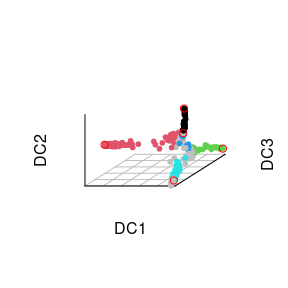# destiny 2.0 brought the Diffusion Pseudo Time (DPT) class

set.seed(1)

Diffusion Pseudo Time (DPT) is a pseudo time metric based on the transition probability of a diffusion process (Haghverdi et al. 2016).

destiny supports DPT in addition to its primary function of creating DiffusionMaps from data.

library(destiny)    # load destiny…
data(guo)           # …and sample data
library(gridExtra)  # Also we need grid.arrange

DPT is in practice independent of Diffusion Maps:

par(mar = rep(0, 4))
graph <- igraph::graph_from_literal(
data -+ 'transition probabilities' -+ DiffusionMap,
'transition probabilities' -+ DPT)
plot(
graph, layout = igraph::layout_as_tree,
vertex.size = 50,
vertex.color = 'transparent',
vertex.frame.color = 'transparent',
vertex.label.color = 'black')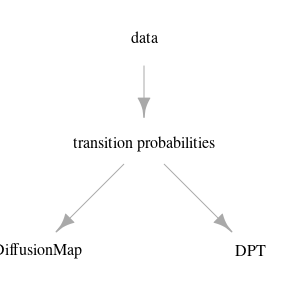However in order not to overcomplicate things, in destiny, you have to create DPT objects from DiffusionMap objects.
(If you really only need the DPT, skip Diffusion Component creation by specifying n_eigs = 0)

dm <- DiffusionMap(guo)
dpt <- DPT(dm)

The resulting object of a call like this will have three automatically chosen tip cells. You can also specify tip cells:

set.seed(4)
dpt_random <- DPT(dm, tips = sample(ncol(guo), 3L))

Plotting without parameters results in the DPT of the first root cell:

TODO: wide plot

grid.arrange(plot(dpt), plot(dpt_random), ncol = 2)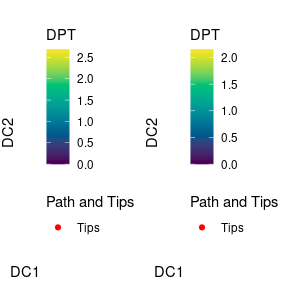Other possibilities include the DPT from the other tips or everything supported by plot.DiffusionMap:

TODO: wide plot

grid.arrange(
plot(dpt, col_by = 'DPT3'),
plot(dpt, col_by = 'Gata4', pal = viridis::magma),
ncol = 2
)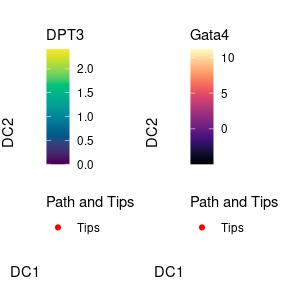The DPT object also contains a clustering based on the tip cells and DPT, and you can specify where to draw paths from and to:

plot(dpt, root = 2, paths_to = c(1,3), col_by = 'branch')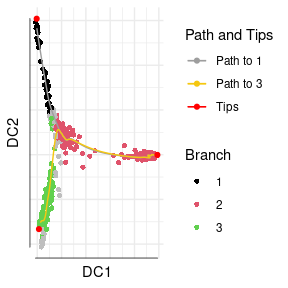You can further divide branches. First simply plot branch colors like we did above, then identify the number of the branch you intend to plot, and then specify it in a subsequent plot call. In order to see the new branches best, we specify a dcs argument that visually spreads out out all four branches.

plot(dpt, col_by = 'branch', divide = 3, dcs = c(-1,-3,2), pch = 20)